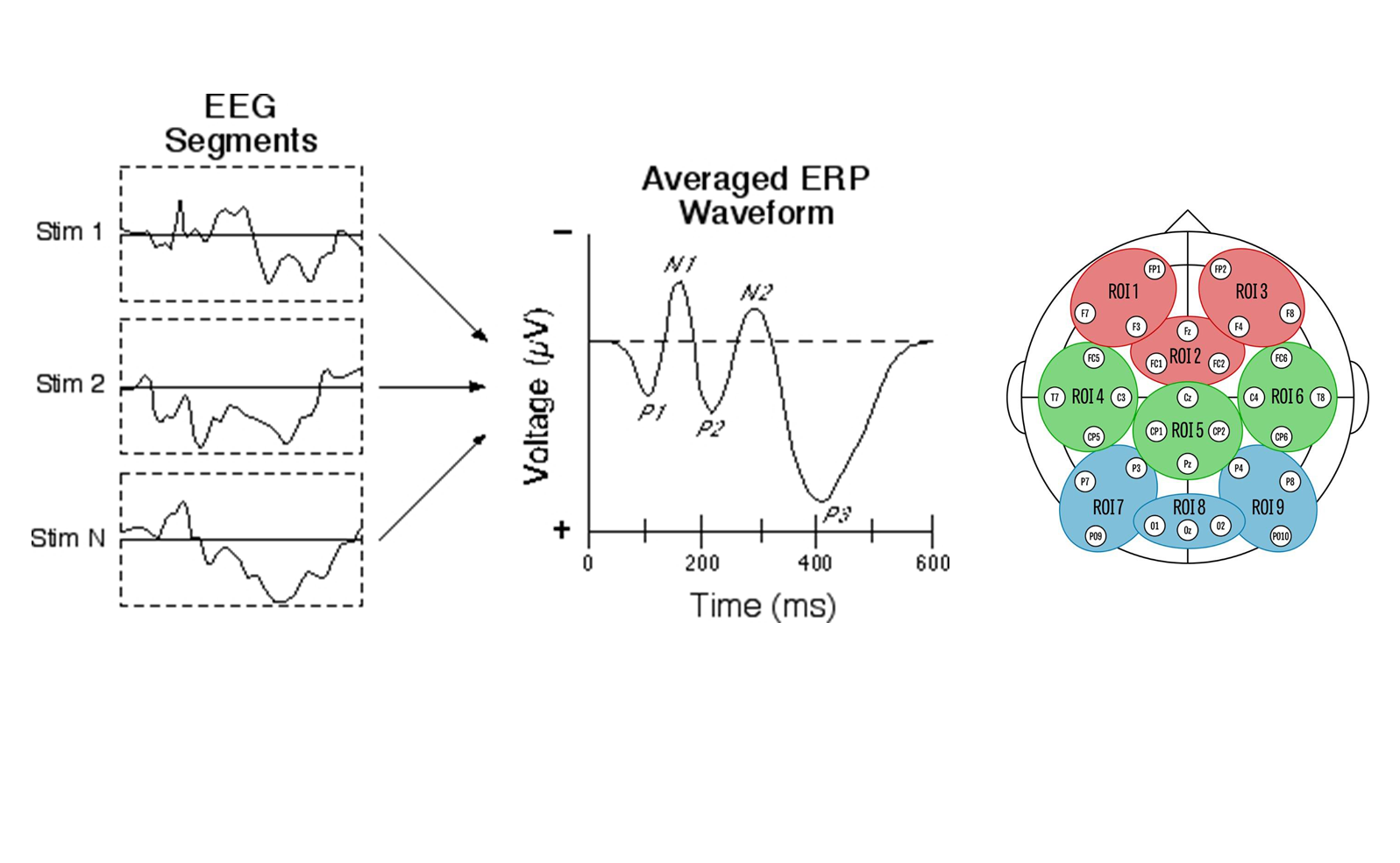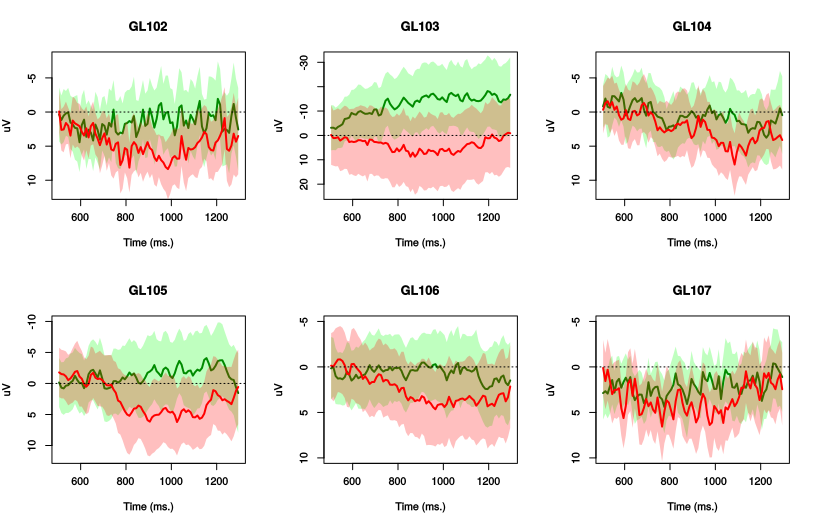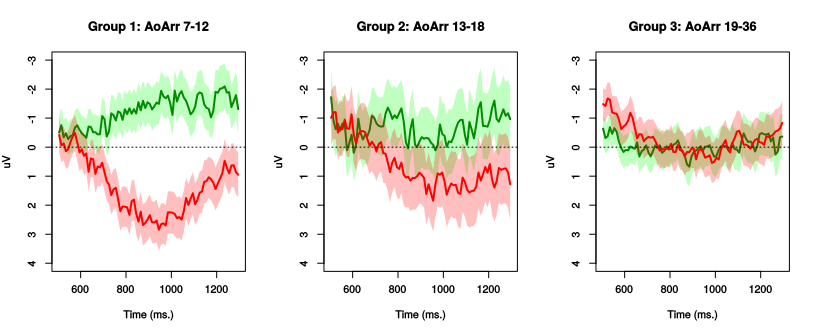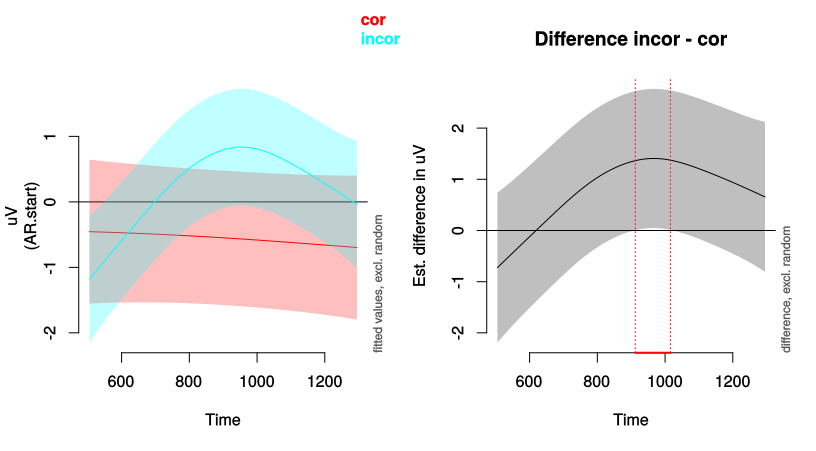Generalized additive models for EEG data

Martijn Wieling
University of Groningen

This lecture

• Introduction
• ERPs to study grammatical gender violations
• Research question
• Design
• Methods (R code) and results
• Discussion

ERPs to study grammatical gender violations

• A P600 (a positivity 'around' 600 ms. after stimulus onset) is sensitive to grammatical violations
• An N400 (a negativity 'around' 400 ms. after stimulus onset) is modulated by semantic context and lexical properties of a word
• The P600/N400 are found by comparing incorrect to correct sentences
• Native speakers appear to show a P600 for grammatical gender violations
• But analyzed by averaging over items and over subjects!

This study

• In this study we are interested in how non-native speakers respond to grammatical gender violations (joint work with Nienke Meulman)
• Grammatical gender is very hard to learn for L2 learners
• Even though behaviorally L2 learners might show correct responses, the brain may reveal differences in processing grammatical gender

Research question

• Is the P600 for grammatical gender violations dependent on age of arrival for the L2 learners of German?

ERP data

• Today: analysis of single region of interest (ROI 8)Design

• 67 L2 speakers of German (Slavic L1)
• Auditory presentation of correct sentences or sentences with a grammatical gender violation (incorrect determiner; no determiners in L1)
• 48 items in each condition: 96 trials per participant (minus artifacts)
• Example:

Nach der Schlägerei ist das/*der Auge des Angestellten von der Krankenschwester versorgt worden.

[After the fight theneut/*themasc eye of the worker was treated by the nurse]

Data overview

dat = dat[order(dat\$Subject, dat\$TrialNr, dat\$Time), ]  # sort data per trial
dat\$start.event <- dat\$Time == min(dat\$Time)  # mark the start of every new trial
#        uV Time Subject Word TrialNr  Type AoArr start.event
# 721  8.94  505   GL102 Wald       2 incor     8        TRUE
# 722 15.56  515   GL102 Wald       2 incor     8       FALSE
# 723 21.31  525   GL102 Wald       2 incor     8       FALSE
# 724 13.32  535   GL102 Wald       2 incor     8       FALSE
# 725 19.11  545   GL102 Wald       2 incor     8       FALSE
# 726 17.96  555   GL102 Wald       2 incor     8       FALSE
dim(dat)  # signal was downsampled to 100 Hz
#  442160      8

Much individual variationGeneral patterns exist

(note the arbitrary age splits, however)Investigating difference between correct and incorrect

(R version 4.1.0 (2021-05-18), mgcv version 1.8.36, itsadug version 2.4)

library(mgcv)

# duration discrete=F: 3600 s.; 1/2/4/8/16 threads: 1000/560/300/200/250 s.
system.time(m0 <- bam(uV ~ s(Time, by = Type) + Type + s(Time, Subject, by = Type,
bs = "fs", m = 1) + s(Time, Word, by = Type, bs = "fs", m = 1), data = dat,
rho = rhoval, AR.start = dat\$start.event, discrete = T, nthreads = 8))
#    user  system elapsed
#    1088    2948     289
• Time window was set to [500,1300] to limit CPU time
• ACF of model without rho was used to determine rhoval: 0.91
• Note that the difference between correct and incorrect will be overly conservative

Global difference between correct and incorrect

summary(m0)  # slides only show the relevant part of the summary
# Parametric coefficients:
#             Estimate Std. Error t value Pr(>|t|)
# (Intercept)   -0.561      0.521   -1.08    0.282
# Typeincor      0.803      0.670    1.20    0.231
#
# Approximate significance of smooth terms:
#                             edf Ref.df    F  p-value
# s(Time):Typecor            1.11   1.20 0.24    0.635
# s(Time):Typeincor          3.32   4.32 6.77 1.65e-05 ***
# s(Time,Subject):Typecor   58.99 603.00 0.90   <2e-16 ***
# s(Time,Subject):Typeincor 53.97 602.00 0.48   <2e-16 ***
# s(Time,Word):Typecor      68.31 864.00 0.29   <2e-16 ***
# s(Time,Word):Typeincor    65.86 863.00 0.26   <2e-16 ***
#
# Deviance explained = 5.2%

Visualizing difference between correct and incorrect

plot_smooth(m0, view = "Time", rug = F, plot_all = "Type", main = "")
plot_diff(m0, view = "Time", comp = list(Type = c("incor", "cor")))  # overly conservativeModeling the difference directly using a binary curve

dat\$IsIncorrect <- (dat\$Type == "incor") * 1  # create binary predictor: 0 = cor, 1 = incor
m0b <- bam(uV ~ s(Time) + s(Time, by = IsIncorrect) + s(Time, Subject, bs = "fs",
m = 1) + s(Time, Subject, by = IsIncorrect, bs = "fs", m = 1) + s(Time, Word,
bs = "fs", m = 1) + s(Time, Word, by = IsIncorrect, bs = "fs", m = 1), data = dat,
rho = rhoval, AR.start = dat\$start.event, discrete = T, nthreads = 8)
• s(Time, by=IsIncorrect) is equal to 0 whenever IsIncorrect equals 0
• Correct case: s(Time) + 0 = s(Time)
• Incorrect case: s(Time) + s(Time, by=IsIncorrect)
• Difference between correct and incorrect: s(Time, by=IsIncorrect)
• Binary curve difference is non-centered (i.e. includes intercept difference)
• This approach is not overly conservative, as the dependency between the nonlinear patterns for the correct and incorrect case per subject (and word) in the random effects is explicitly included (Sóskuthy, 2021)

Results using a binary curve

summary(m0b, re.test = FALSE)  # summary without random effects (quicker to compute)
# Parametric coefficients:
#             Estimate Std. Error t value Pr(>|t|)
# (Intercept)   -0.573      0.468   -1.22    0.221
#
# Approximate significance of smooth terms:
#                      edf Ref.df   F p-value
# s(Time)             1.64   2.05 0.6   0.535
# s(Time):IsIncorrect 4.08   5.00 3.9   0.002 **
• s(Time):IsIncorrect shows the significance of the combined intercept and non-linear difference between correct and incorrect

Modeling the difference using an ordered factor

dat\$TypeO <- as.ordered(dat\$Type)  # creating an ordered factor ...
contrasts(dat\$TypeO) <- "contr.treatment"  # ... with contrast treatment: cor = 0, incor = 1
m0o <- bam(uV ~ s(Time) + s(Time, by = TypeO) + TypeO + s(Time, Subject, bs = "fs",
m = 1) + s(Time, Subject, by = TypeO, bs = "fs", m = 1) + s(Time, Word, bs = "fs",
m = 1) + s(Time, Word, by = TypeO, bs = "fs", m = 1), data = dat, rho = rhoval,
AR.start = dat\$start.event, discrete = T, nthreads = 8)
• s(Time, by=TypeO) is equal to 0 whenever TypeO equals cor (reference level)
• Difference between correct and incorrect: s(Time, by=TypeO) + TypeO
• s(Time, by=TypeO): centered non-linear difference
• TypeO (must be included): intercept difference
• The random-effects specification is effectively the same as that of the binary curve model, given that factor smooths involving ordered factors are not centered
• This random reference/difference smooths approach (Sóskuthy, 2021) is appropriate and not overly conservative

Results using an ordered factor

summary(m0o, re.test = FALSE)
# Parametric coefficients:
#             Estimate Std. Error t value Pr(>|t|)
# (Intercept)   -0.573      0.468   -1.22    0.221
# TypeOincor     0.789      0.575    1.37    0.170
#
# Approximate significance of smooth terms:
#                     edf Ref.df    F p-value
# s(Time)            1.64   2.05 0.60   0.535
# s(Time):TypeOincor 3.08   4.00 4.58   0.001 **
• The \(p\)-value of the parametric coefficient TypeOincor represents the significance of the intercept difference between correct and incorrect
• The \(p\)-value of the smooth term s(Time):TypeOincor represents the significance of the non-linear difference between correct and incorrect

Visualization of both difference curves

plot(m0b, select = 2, shade = T, rug = F, main = "Binary difference", ylim = c(-3, 3))
plot(m0o, select = 2, shade = T, rug = F, main = "Ordered factor difference", ylim = c(-3, 3))